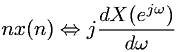Equations > Signal Processing > Fourier Transform Properties > Discrete-Time Fourier frequency differentiation property

### Discrete-Time Fourier frequency differentiation propertyLatex Code:

MathML Code:

 $\mathrm{nx}\left(n\right)⇔j\frac\mathrm{dX}\left({e}^{j\omega }\right)d\omega$

MathType 5.0: